# Darboux-Baire-1-function

(diff) ← Older revision | Latest revision (diff) | Newer revision → (diff)

Darboux–Baire one-function, Darboux function of the first Baire class

A real-valued function of a real variable of the first Baire class (cf. Baire classes) that satisfies the Darboux property.

In the first Baire class, the Darboux property is known to be equivalent to other properties. For example, in 1907, J. Young considered [a19] the following property: For eachthere exist sequences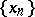,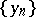such thatand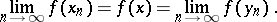He proved that for functions of the first Baire class, the Darboux property and this Young property are equivalent. In 1922, K. Kuratowski and W. Sierpiński proved [a10] that for real-valued functions of the first Baire class and defined on an interval, the Darboux property is equivalent to the fact that the function has a connected graph (cf. also Graph of a mapping). In 1974, J. Brown showed [a3] that for real functions of the first Baire class and defined on an interval, the Darboux property is equivalent to Stallings almost continuity. In 1988, it was shown [a4] that for a functionof the first Baire class, the Darboux property ofis equivalent to extendibility of. In 1995, it was proved [a7] that a functionin the first Baire class is a Darboux function if and only ifis first return continuous.

The set of all Darboux functions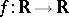of the first Baire class will be denoted by. The classcontains many important classes of functions, for example the classof all (finite) derivatives, the classof all Stallings almost-continuous functions, and the classof all approximately continuous functions (cf. also Approximate continuity). For bounded functions (denoted by the prefix),One can prove [a5] that in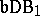(with the metric of the uniform convergence) the sets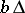and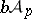are very small, in fact, they are superporous at each point of. I. Maximoff proved ([a12], [a13], [a11]) that each function from the larger class (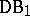) can be transformed into a function from the smaller class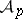(or) by a suitable homeomorphic change of variables. In 1961, C. Goffman and D. Waterman considered [a9] connections betweenandfor functions mapping a Euclidean space into a metric space.

In 1950, Z. Zahorski considered [a20] the following hierarchy of classes of functions:Each of these classes is defined in terms of an associated set of a function (the associated sets ofare all sets of the form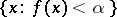and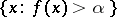). The two largest classes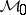and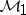are equal to, the smallest class (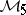) is equal to. Zahorski also proved that the classfits into this "sequence of classes of functions" (if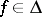, thenand if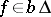; then). The similar hierarchy of classes of functions of two variables has been considered in [a14], [a15], [a18].

The classis closed with respect to uniform convergence. The maximal additive family foris the class of all continuous functions. The maximal multiplicative family foris the class of Darboux functionswith the property: Ifis a right-hand (left-hand) discontinuity point of, thenand there is a sequencesuch that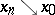(respectively,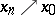) and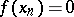, [a8]. Of course,does not form a ring, but for each functionthere exists a ring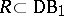containing the class of all continuous functions and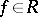(see, e.g., [a18]).

In 1963, H. Croft constructed [a6] a functionthat is zero almost-everywhere but not identically zero. In 1974, a general method for constructing such functions was given ([a1], [a2]): Let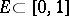be an-set (cf. also Set of type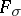(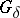)) that is bilaterally c-dense-in-itself. Then there exists a functionsuch that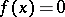for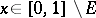andfor all.

Except the standard class, one can also consider the class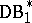(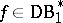ifis a Darboux function and for every non-empty closed setthere is an open intervalsuch that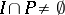and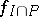is continuous; see, e.g., [a17]).

How to Cite This Entry:
Darboux-Baire-1-function. Encyclopedia of Mathematics. URL: http://encyclopediaofmath.org/index.php?title=Darboux-Baire-1-function&oldid=18540
This article was adapted from an original article by R.J. Pawlak (originator), which appeared in Encyclopedia of Mathematics - ISBN 1402006098. See original article